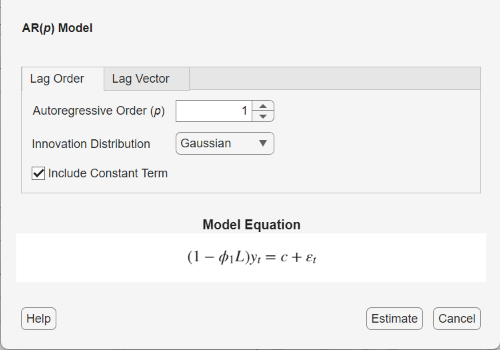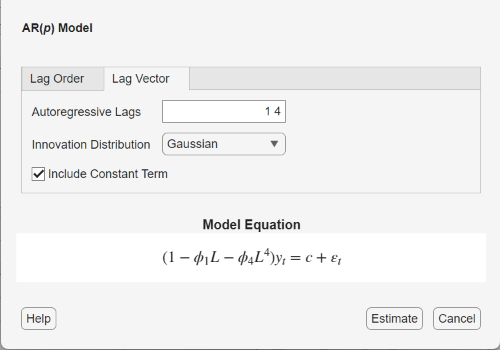AR Model Specifications

Default AR Model

This example shows how to use the shorthand arima(p,D,q) syntax to specify the default AR($p$) model,

${y}_{t}=c+{\varphi }_{1}{y}_{t-1}+\dots +{\varphi }_{p}{y}_{t-p}+{\epsilon }_{t}.$

By default, all parameters in the created model object have unknown values, and the innovation distribution is Gaussian with constant variance.

Specify the default AR(2) model:

Mdl = arima(2,0,0)
Mdl =
arima with properties:

Description: "ARIMA(2,0,0) Model (Gaussian Distribution)"
Distribution: Name = "Gaussian"
P: 2
D: 0
Q: 0
Constant: NaN
AR: {NaN NaN} at lags [1 2]
SAR: {}
MA: {}
SMA: {}
Seasonality: 0
Beta: [1×0]
Variance: NaN

The output shows that the created model object, Mdl, has NaN values for all model parameters: the constant term, the AR coefficients, and the variance. You can modify the created model object using dot notation, or input it (along with data) to estimate.

AR Model with No Constant Term

This example shows how to specify an AR(p) model with constant term equal to zero. Use name-value syntax to specify a model that differs from the default model.

Specify an AR(2) model with no constant term,

${y}_{t}={\varphi }_{1}{y}_{t-1}+{\varphi }_{2}{y}_{t-2}+{\epsilon }_{t},$

where the innovation distribution is Gaussian with constant variance.

Mdl = arima('ARLags',1:2,'Constant',0)
Mdl =
arima with properties:

Description: "ARIMA(2,0,0) Model (Gaussian Distribution)"
Distribution: Name = "Gaussian"
P: 2
D: 0
Q: 0
Constant: 0
AR: {NaN NaN} at lags [1 2]
SAR: {}
MA: {}
SMA: {}
Seasonality: 0
Beta: [1×0]
Variance: NaN

The ARLags name-value argument specifies the lags corresponding to nonzero AR coefficients. The property Constant in the created model object is equal to 0, as specified. The model object has default values for all other properties, including NaN values as placeholders for the unknown parameters: the AR coefficients and scalar variance.

You can modify the created model object using dot notation, or input it (along with data) to estimate.

AR Model with Nonconsecutive Lags

This example shows how to specify an AR(p) model with nonzero coefficients at nonconsecutive lags.

Specify an AR(4) model with nonzero AR coefficients at lags 1 and 4 (and no constant term),

${y}_{t}=0.2+0.8{y}_{t-1}-0.1{y}_{t-4}+{\epsilon }_{t},$

where the innovation distribution is Gaussian with constant variance.

Mdl = arima('ARLags',[1,4],'Constant',0)
Mdl =
arima with properties:

Description: "ARIMA(4,0,0) Model (Gaussian Distribution)"
Distribution: Name = "Gaussian"
P: 4
D: 0
Q: 0
Constant: 0
AR: {NaN NaN} at lags [1 4]
SAR: {}
MA: {}
SMA: {}
Seasonality: 0
Beta: [1×0]
Variance: NaN

The output shows the nonzero AR coefficients at lags 1 and 4, as specified. The property P is equal to 4, the number of presample observations needed to initialize the AR model. The unconstrained parameters are equal to NaN.

Display the value of AR:

Mdl.AR
ans=1×4 cell array
{[NaN]}    {}    {}    {[NaN]}

The AR cell array returns four elements. The first and last elements (corresponding to lags 1 and 4) have value NaN, indicating these coefficients are nonzero and need to be estimated or otherwise specified by the user. arima sets the coefficients at interim lags equal to zero to maintain consistency with MATLAB® cell array indexing.

ARMA Model with Known Parameter Values

This example shows how to specify an ARMA(p, q) model with known parameter values. You can use such a fully specified model as an input to simulate or forecast.

Specify the ARMA(1,1) model

${y}_{t}=0.3+0.7\varphi {y}_{t-1}+{\epsilon }_{t}+0.4{\epsilon }_{t-1},$

where the innovation distribution is Student's t with 8 degrees of freedom, and constant variance 0.15.

tdist = struct('Name','t','DoF',8);
Mdl = arima('Constant',0.3,'AR',0.7,'MA',0.4,...
'Distribution',tdist,'Variance',0.15)
Mdl =
arima with properties:

Description: "ARIMA(1,0,1) Model (t Distribution)"
Distribution: Name = "t", DoF = 8
P: 1
D: 0
Q: 1
Constant: 0.3
AR: {0.7} at lag 
SAR: {}
MA: {0.4} at lag 
SMA: {}
Seasonality: 0
Beta: [1×0]
Variance: 0.15

All parameter values are specified, that is, no object property is NaN-valued.

AR Model with t Innovation Distribution

This example shows how to specify an AR($p$) model with a Student's t innovation distribution.

Specify an AR(2) model with no constant term,

${y}_{t}={\varphi }_{1}{y}_{t-1}+{\varphi }_{2}{y}_{t-2}+{\epsilon }_{t},$

where the innovations follow a Student's t distribution with unknown degrees of freedom.

Mdl = arima('Constant',0,'ARLags',1:2,'Distribution','t')
Mdl =
arima with properties:

Description: "ARIMA(2,0,0) Model (t Distribution)"
Distribution: Name = "t", DoF = NaN
P: 2
D: 0
Q: 0
Constant: 0
AR: {NaN NaN} at lags [1 2]
SAR: {}
MA: {}
SMA: {}
Seasonality: 0
Beta: [1×0]
Variance: NaN

The value of Distribution is a struct array with field Name equal to 't' and field DoF equal to NaN. The NaN value indicates the degrees of freedom are unknown, and need to be estimated using estimate or otherwise specified by the user.

Specify AR Model Using Econometric Modeler App

In the Econometric Modeler app, you can specify the lag structure, presence of a constant, and innovation distribution of an AR(p) model by following these steps. All specified coefficients are unknown, estimable parameters.

1. At the command line, open the Econometric Modeler app.

econometricModeler

Alternatively, open the app from the apps gallery (see Econometric Modeler).

2. In the Time Series pane, select the response time series to which the model will be fit.

3. On the Econometric Modeler tab, in the Models section, click .

The AR Model Parameters dialog box appears.4. Specify the lag structure. To specify an AR(p) model that includes all AR lags from 1 through p, use the Lag Order tab. For the flexibility to specify the inclusion of particular lags, use the Lag Vector tab. For more details, see Specifying Lag Operator Polynomials Interactively. Regardless of the tab you use, you can verify the model form by inspecting the equation in the Model Equation section.

For example:

• To specify an AR(2) model that includes a constant, includes the first lag, and has a Gaussian innovation distribution, set Autoregressive Order to 2.

• To specify an AR(2) model that includes the first lag, has a Gaussian distribution, but does not include a constant:

1. Set Autoregressive Order to 2.

2. Clear the Include Constant Term check box.

• To specify an AR(4) model containing nonconsecutive lags

${y}_{t}={\varphi }_{1}{y}_{t-1}+{\varphi }_{4}{y}_{t-4}+{\epsilon }_{t},$

where εt is a series of IID Gaussian innovations:

1. Click the Lag Vector tab.

2. Set Autoregressive Lags to 1 4.

3. Clear the Include Constant Term check box.• To specify an AR(2) model that includes the first lag, includes a constant term, and has t-distributed innovations:

1. Set Autoregressive Lags to 2.

2. Click the button, then select t.

The degrees of freedom parameter of the t distribution is an unknown but estimable parameter.

After you specify a model, click to estimate all unknown parameters in the model.